On Gravitational Magnetic Field Derived from Special Relativity Leading to Dark Matter Force

Delso J

Bachelor's Degree in Physics by Zaragoza University, Spain

Article
Article Info
Author Info
Figures & Data

Abstract

The gravitational magnetic force is derived from Special Relativity force transformations. Energy associated with this new force is stored in the dark matter energy tensor D (4, 0) related to the Riemann curvature.

Dark matter gravity is generated by D(4,0) energy related directly to the S(4,0) tensor, however this gravity is attributed to exotic particles never detected, galaxies in our universe are rotating with such speed that the gravity generated by their observable matter could not possibly hold them together.

Total Energy T (4, 0) and energy tensors are denied to complete the General Relativity field equations. The Ricci decomposition is a way of breaking up the Riemann curvature tensor into three orthogonal tensors, Z (4, 0), Weyl tensor C (4, 0) and S (4, 0).

Key Words

Dark Matter, Expansion of the Universe, Riemann Curvature, Ricci Decomposition, Special Relativity, Gravitational Magnetic Field, General Relativity Field Equations

The gravitational magnetic field is derived from Special Relativity force transformations

In S two identical particles without electric charge (q = 0) and mass m are moving with v1x = v2x = u, x1 = x2 = x0, z1 = z2 = 0 and distance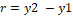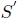is moving with v = u respect to S, inthe particles are at rest with mass m0 and distance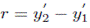since q = 0 the only force is the gravitational force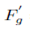.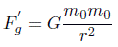In S, gravitational force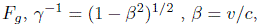c the speed of light.The relativistic transformation of net force is .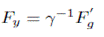So, there is a force, FBg, associated with the gravitational magnetic field Bg and Fy = Fg+ FBg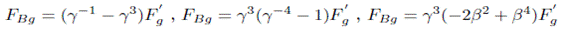With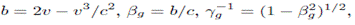transformations are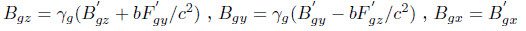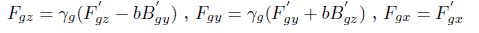FBg is associated with Dark Matter energy which has been stored in the dark matter energy tensor D (4, 0) defined below. You can also see discussions about dark matter and the expansion of the Universe in .

Conformal energy U defined as a combination of C and the Hodge dual of C, dark matter energy D defined as a combination of S and the Hodge dual of S, similar definitions for V/Z and T/R

The Ricci decomposition is a way of breaking up the Riemann curvature tensor into three orthogonal tensors, Z, Weyl tensor C and S, S tensor generates the dark matter gravity [6-8].

Rijkl = Zijkl + Cijkl + Sijkl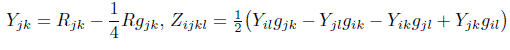where Rabcd is the Riemann tensor, Rab is the Ricci tensor, R is the Ricci scalar (the scalar curvature).

The conformal energy tensor U can be defined as a combination of C and the Hodge dual of C [1-3].The new dark matter energy tensor D can be defined as a combination of S and the Hodge dual of S.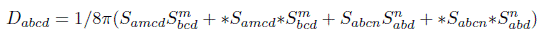The new energy tensor V can be defined as a combination of Z and the Hodge dual of Z.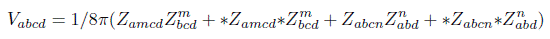The new Total Energy tensor T can be defined as a combination of the Riemann tensor R and the Hodge dual of R.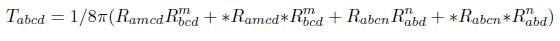Hodge Dual Definitions

The Hodge dual definition for Electromagnetic tensor and Weyl tensor .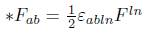The Hodge dual definition for dark matter S tensor, Z and R tensors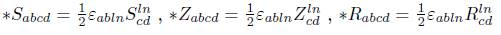Weyl tensor C (4, 0) is related to the new Conformal Energy tensor U (4, 0). Dark matter tensor S (4, 0) is related to the new dark matter energy tensor D (4, 0). Z (4, 0) tensor is related to the new energy tensor V (4, 0). Riemann tensor R (4, 0) is related to the new Total Energy tensor T (4, 0).

Complete General Relativity Field Equations

The complete field equations are described by a new T (4, 0) tensor for Total Energy, the new conformal energy tensor U (4, 0), the new energy tensor V (4, 0) and the new dark matter energy tensor D (4, 0).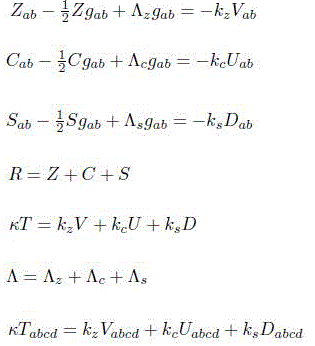In the general theory of relativity the Einstein field equations relate the geometry of spacetime to the distribution of matter .Conclusions

When a charged particle is moving it generates a magnetic field that interacts with charged particles in motion, similarly when a particle with mass is moving it generates a gravitational magnetic field that interacts with other particles in motion.

This new force, the gravitational magnetic force has been detected by astronomers for decades but has been misinterpreted, in a galaxy there are no dark matter particles generating the dark matter force, moving stars generate gravitational magnetic fields that interact with other stars in motion.

The gravitational magnetic force is derived from Special Relativity force transformations, if Special Relativity is correct the gravitational magnetic force is irrefutable.

References

1. Jesus Delso Lapuerta (2020) On the conformal energy tensor defined as a combination of Weyl tensor and the Hodge dual of Weyl tensor Vixra.org Relativity and Cosmology, 2-3.
2. Roger Penrose (2006) El camino a la realidad Random House Mondadori, Barcelona 619.
3. Roger Penrose (2006) El camino a la realidad Random House Mondadori, Barcelona 629.
4. Roger Penrose (2006) El camino a la realidad Random House Mondadori, Barcelona 1255.
5. Albert Einstein (1985) El significado de la relatividad Planeta-De Agos- tini, Barcelona 175.
6. Besse Arthur L (1987) Einstein manifolds, Ergebnisse der Mathematik und ihrer Grenzgebiete (3) [Results in Mathematics and Related Areas (3)], vol. 10, Berlin, New York: Springer-Verlag, pp. xii+510.
7. Sharpe RW (1997) Differential Geometry: Cartan’s Generalization of Klein’s Erlangen Program, Springer-Verlag, New York, ISBN 0-387-94732-9. Section 6.1 discusses the decomposition. Versions of the decomposition also enter into the discussion of conformal and projective geometries, in chapters 7 and 8.
8. Singer IM, Thorpe JA (1969) The curvature of 4-dimensional Einstein spaces, Global Analysis (Papers in Honor of K. Kodaira), Univ. Tokyo Press 355–365.
9. Robert Resnick (1977) Introduction a la Teoria Especial de la Relatividad Editorial Limmusa, Mexico DF 139.
10. Delso J (2021) On Dark Matter Gravity Force Generated by the Expansion of the Universe. OSP J Phy Astronomy 2: JPA-2-111.

Research Article

Publication history

Accepted date: May 04, 2021
Published date: May 15, 2021

©2021 Delso J. This is an open-access article distributed under the terms of the Creative Commons Attribution License, which permits unrestricted use, distribution, and reproduction in any medium, provided the original author and source are credited.

Citation

Delso J. (2021) On Gravitational Magnetic Field Derived from Special Relativity Leading to Dark Matter Force. OSP J Phy Astronomy 2: JPA-2-115

Jesus Delso Lapuerta

Bachelor's Degree in Physics by Zaragoza University, Spain jesus.delso@gmail.com

No Figures